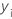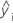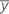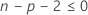# Methods and formulas for goodness-of-fit statistics in Analyze Factorial Design

## S

### Notation

TermDescription
MSEmean square error

## R-sq

R2 is also known as the coefficient of determination.

### Notation

TermDescription
yi i th observed response valuemean responsei th fitted response

## R-sq (adj)

While the calculations for adjusted R2 can produce negative values, Minitab displays zero for these cases.

### Notation

TermDescriptionith observed response valueith fitted responsemean response
nnumber of observations
pnumber of terms in the model

## R-sq (pred)

While the calculations for R2(pred) can produce negative values, Minitab displays zero for these cases.

### Notation

TermDescription
yi i th observed response valuemean response
n number of observations
ei i th residual
hi i th diagonal element of X(X'X)–1X'
X design matrix

## PRESS

Assesses your model's predictive ability and is calculated as:

### Notation

TermDescription
nnumber of observations
eiith residual
hi

ith diagonal element of

X (X' X)-1X'

## R-sq (WP)

In split-plot designs, R2 (WP) is the proportion of variation among whole plots that is accounted for by all of the terms in the model that involve only hard-to-change factors.

### Notation

TermDescription
SS (WPerror)Sum of squares for the whole plot error term
SS Total (WP)Sum of sequential SS for all terms that involve the whole plot error term and only hard-to-change factors

## R-sq(SP)

In split-plot designs, R2 (SP) is the proportion of variation among subplots (within whole plots) that is accounted for by the model.

### Notation

TermDescription
SS (SPerror)sums of squares for the subplot error term
SS Total (SP)SS Total – SS Total(WP)
SS Total (WP)sum of sequential SS for all terms that involve the whole plot error term and only hard-to-change factors

## Log-likelihood

For unweighted analyses, Minitab uses the following equation:
For an analysis that has weights for the observations, Minitab uses the following equation:

Observations with weights of 0 are not in the analysis.

### Notation

TermDescription
nthe number of observations
Rthe sum of squares for error for the model
withe weight of the ith observation

## AICc (Akaike's Corrected Information Criterion)

AICc is not calculated when.

### Notation

TermDescription
nthe number of observations
pthe number of coefficients in the model, including the constant

## BIC (Bayesian Information Criterion)

### Notation

TermDescription
pthe number of coefficients in the model, including the constant
nthe number of observations

## Mallows' Cp

### Notation

TermDescription
SSEpsum of squared errors for the model under consideration
MSEmmean square error for the model with all candidate terms
nnumber of observations
pnumber of terms in the model, including the constant
By using this site you agree to the use of cookies for analytics and personalized content.  Read our policy